# 数据挖掘 第四篇：OLS回归分析

OLS（最小二乘法）主要用于线性回归的参数估计，它的思路很简单，就是求一些使得实际值和模型估值之差的平方和达到最小的值，将其作为参数估计值。就是说，通过最小化误差的平方和寻找数据的最佳函数匹配。利用最小二乘法可以简便地求得未知的数据，并使得这些求得的数据与实际数据之间误差的平方和为最小。最小二乘法可用于曲线拟合，其他一些优化问题也可通过最小化能量或最大化熵用最小二乘法来表达。

## 一，OLS回归

OLS法通过一系列的预测变量来预测响应变量（也可以说是在预测变量上回归响应变量）。线性回归是指对参数β为线性的一种回归（即参数只以一次方的形式出现）模型：

Yt=α+βxtt （t=1……n）表示观测数

• Yt  被称作因变量
• xt  被称作自变量
• α、β 为需要最小二乘法去确定的参数，或称回归系数
• μt  为随机误差项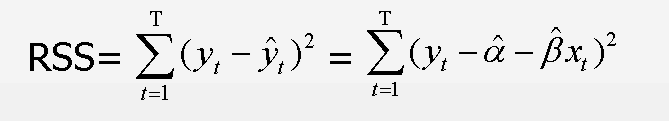• 正态性：对于固定的自变量值，因变量值成正太分布
• 独立性：个体之间相互独立
• 线性相关：因变量和自变量之间为线性相关
• 同方差性：因变量的方差不随自变量的水平不同而变化，即因变量的方差是不变的

## 二，用lm()拟合回归模型

lm(formula, data)

formula中的符号注释：

• ~ 分割符号，左边为因变量，右边为自变量，例如， z~x+y，表示通过x和y来预测z
• + 分割预测变量
• : 表示预测变量的交互项，例如，z~x+y+x:y
• * 表示所有可能的交互项，例如，z~x*y 展开为 z~x+y+x:y
• ^ 表示交互项的次数，例如，z ~ (x+y)^2，展开为z~x+y+x:y
• . 表示包含除因变量之外的所有变量，例如，如果只有三个变量x，y和z，那么代码 z~. 展开为z~x+y+x:y
• -1 删除截距项，强制回归的直线通过原点
• I() 从算术的角度来解释括号中的表达式，例如，z~y+I(x^2) 表示的拟合公式是 z=a+by+cx2
• function 可以在表达式中应用数学函数，例如，log(z) ~x+y

• summary() 展示拟合模型的详细结果
• coefficients() 列出捏模型的参数（截距项intercept和斜率）
• confint() 提供模型参数的置信区间
• residuals() 列出拟合模型的残差值
• fitted() 列出拟合模型的预测值
• anova() 生成一个拟合模型的方差分析表
• predict() 用拟合模型对新的数据预测响应变量

1，线性拟合

fit <- lm(weight~height,data=women)

> summary(fit)

Call:
lm(formula = weight ~ height, data = women)

Residuals:
Min      1Q  Median      3Q     Max
-1.7333 -1.1333 -0.3833  0.7417  3.1167

Coefficients:
Estimate Std. Error t value Pr(>|t|)
(Intercept) -87.51667    5.93694  -14.74 1.71e-09 ***
height        3.45000    0.09114   37.85 1.09e-14 ***
---
Signif. codes:  0 ‘***’ 0.001 ‘**’ 0.01 ‘*’ 0.05 ‘.’ 0.1 ‘ ’ 1

Residual standard error: 1.525 on 13 degrees of freedom
Multiple R-squared:  0.991,    Adjusted R-squared:  0.9903
F-statistic:  1433 on 1 and 13 DF,  p-value: 1.091e-14

R的平方项（Multiple R-squared）：表明模型可以解释体重99.1%的方差，是实际和预测值之间相关系数的平方。

F统计量（F-statistic）：检验所有的预测变量预测响应变量是否都在某个概率水平之上，

Weight= - 87.51667 + 3.45 * Height

> plot(women$height, women$weight,xlab='height',ylab='weight')
> abline(fit)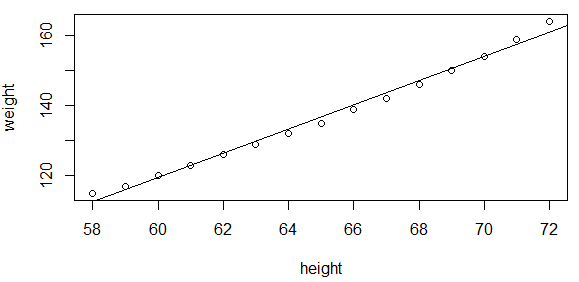2，多项式拟合

> fit2 <- lm(weight~I(height^2),data=women)
> plot(women$height, women$weight,xlab='height',ylab='weight')
> lines(women\$height,fitted(fit2))
> summary(fit2)

Call:
lm(formula = weight ~ I(height^2), data = women)

Residuals:
Min      1Q  Median      3Q     Max
-1.2562 -0.7636 -0.1837  0.4622  2.2654

Coefficients:
Estimate Std. Error t value Pr(>|t|)
(Intercept) 2.390e+01  2.109e+00   11.34 4.12e-08 ***
I(height^2) 2.659e-02  4.926e-04   53.98  < 2e-16 ***
---
Signif. codes:  0 ‘***’ 0.001 ‘**’ 0.01 ‘*’ 0.05 ‘.’ 0.1 ‘ ’ 1

Residual standard error: 1.072 on 13 degrees of freedom
Multiple R-squared:  0.9956,    Adjusted R-squared:  0.9952
F-statistic:  2913 on 1 and 13 DF,  p-value: < 2.2e-16

## 三，回归诊断（标准方法）

回归诊断用于评价回归模型的拟合程度，模型返回的参数多大程度上匹配原始数据。

根据OLS回归的统计假设来评价模型的拟合情况，对于lm()拟合的模型对象，使用plot()函数生成评价模型拟合情况的四幅图形，

> fit <- lm(weight~height,data=women)
> par(mfrow=c(2,2))
> plot(fit)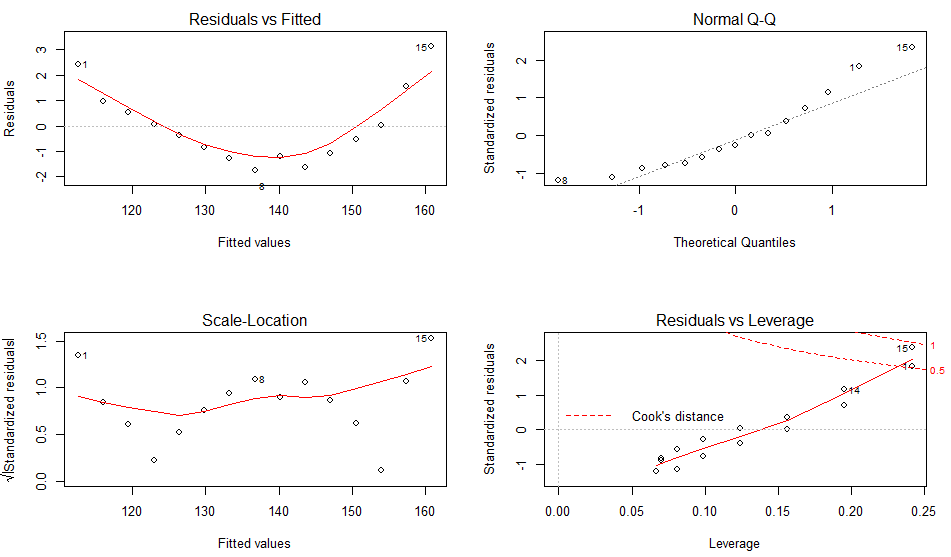• 离群点：表明拟合回归模型对其预测效果不佳（产生巨大的残差）
• 高杠杆点：指自变量因子空间中的离群点（异常值），由许多异常的自变量值组合起来的，与因变量没有关系。
• 强影响点：表明它对模型参数的估计产生的影响过大，非常不成比例。强影响点可以通过Cook距离（Cook distance）统计量来前别。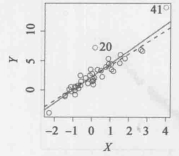## 四，回归诊断（car包）

car包提供了大量的函数，大大增强了拟合和评价回归模型的能力

1，正态性

qqplot()函数提供了精确的正态假设检验方法，

> library(carData)
> library(car)
> par(mfrow=c(1,2))
> fit <- lm(weight~height,data=women)
> qqPlot(fit,labels=row.names(women),id.method='identity',simulate=TRUE,main='qq-fit')
  1 15
> fit2 <- lm(weight~height+I(height^2),data=women)
> qqPlot(fit2,labels=row.names(women),id.method='identity',simulate=TRUE,main='qq-fit2')
 13 15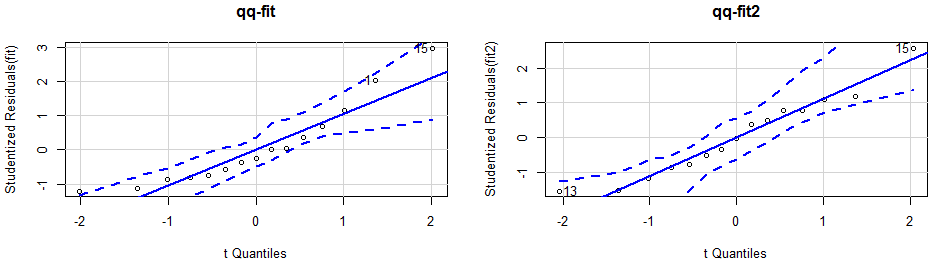2，误差的独立性

car包提供了durbinWatsonTest()函数，用于做Durbin-Watson检验，检测误差的序列相关性。

> durbinWatsonTest(fit)
lag Autocorrelation D-W Statistic p-value
1        0.585079     0.3153804       0
Alternative hypothesis: rho != 0

p值 （p=0）不显著，误差项之间独立

3，线性相关性

crPlots(fit)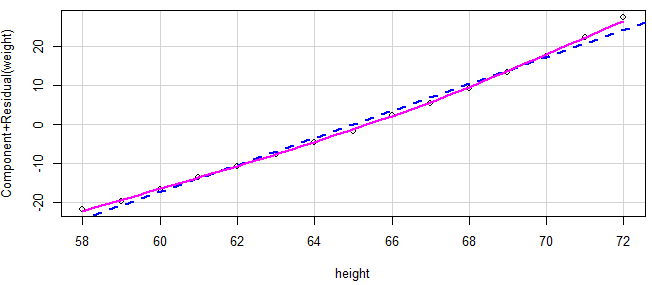4，同方差性

> ncvTest(fit)
Non-constant Variance Score Test
Variance formula: ~ fitted.values
Chisquare = 0.8052115, Df = 1, p = 0.36954

Suggested power transformation:  -0.8985826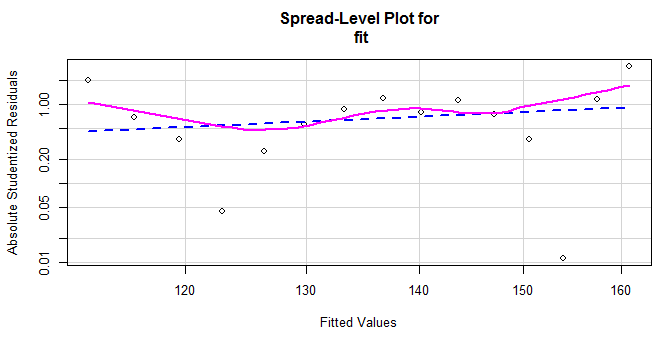## 五，回归诊断（gvlma包）

gvlma()函数，用于对线性模型假设进行综合验证，同事还能验证偏斜度，峰度和异方差的评价。从输出项中可以看出，假设检验的显著性水平是5%。如果p<0.05，说明违反了假设条件。从Global Stat 的Decision 栏中，可以看到数据满足OLS回归模型所有的统计假设（p=0.597）。

> library(gvlma)
> gvmodel <- gvlma(fit)
> summary(gvmodel)
.................
ASSESSMENT OF THE LINEAR MODEL ASSUMPTIONS
USING THE GLOBAL TEST ON 4 DEGREES-OF-FREEDOM:
Level of Significance =  0.05

Call:
gvlma(x = fit)

Value   p-value                   Decision
Global Stat        16.5866 0.0023251 Assumptions NOT satisfied!
Skewness            1.5577 0.2119999    Assumptions acceptable.
Kurtosis            0.1019 0.7496131    Assumptions acceptable.
Link Function      14.1218 0.0001713 Assumptions NOT satisfied!
Heteroscedasticity  0.8052 0.3695398    Assumptions acceptable.

posted @ 2018-12-29 16:53  悦光阴  阅读(45705)  评论(0编辑  收藏  举报